## ThinkDSP¶

This notebook contains code examples from Chapter 8: Filtering and Convolution

In :
# Get thinkdsp.py

import os

if not os.path.exists('thinkdsp.py'):
!wget https://github.com/AllenDowney/ThinkDSP/raw/master/code/thinkdsp.py

In :
import numpy as np
import matplotlib.pyplot as plt

from thinkdsp import decorate

In :
# suppress scientific notation for small numbers
np.set_printoptions(precision=3, suppress=True)


### Smoothing¶

As the first example, I'll look at daily closing stock prices for Facebook, from its IPO on 2012-05-18 to 2020-03-27 (note: the dataset includes only trading days )

In :
if not os.path.exists('FB.csv'):
!wget https://github.com/AllenDowney/ThinkDSP/raw/master/code/FB.csv

In :
import pandas as pd


Out:
Date Open High Low Close Adj Close Volume
0 2012-05-18 42.049999 45.000000 38.000000 38.230000 38.230000 573576400
1 2012-05-21 36.529999 36.660000 33.000000 34.029999 34.029999 168192700
2 2012-05-22 32.610001 33.590000 30.940001 31.000000 31.000000 101786600
3 2012-05-23 31.370001 32.500000 31.360001 32.000000 32.000000 73600000
4 2012-05-24 32.950001 33.209999 31.770000 33.029999 33.029999 50237200
In :
df.tail()

Out:
Date Open High Low Close Adj Close Volume
1972 2020-03-23 149.660004 152.309998 142.250000 148.100006 148.100006 29830800
1973 2020-03-24 155.210007 161.309998 152.570007 160.979996 160.979996 30440400
1974 2020-03-25 158.919998 162.990005 153.059998 156.210007 156.210007 35184300
1975 2020-03-26 158.250000 164.000000 157.020004 163.339996 163.339996 26556800
1976 2020-03-27 158.199997 160.089996 154.750000 156.789993 156.789993 24861900

Extract the close prices and days since start of series:

In :
close = df['Close']
dates = df['Date']
days = (dates - dates) / np.timedelta64(1,'D')


Make a window to compute a 30-day moving average and convolve the window with the data. The valid flag means the convolution is only computed where the window completely overlaps with the signal.

In :
M = 30
window = np.ones(M)
window /= sum(window)
smoothed = np.convolve(close, window, mode='valid')
smoothed_days = days[M//2: len(smoothed) + M//2]


Plot the original and smoothed signals.

In :
plt.plot(days, close, color='gray', alpha=0.6, label='daily close')
plt.plot(smoothed_days, smoothed, color='C1', alpha=0.6, label='30 day average')

decorate(xlabel='Time (days)', ylabel='Price ($)')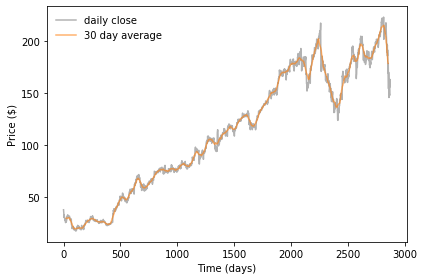## Smoothing sound signals¶ Generate a 440 Hz sawtooth signal. In : from thinkdsp import SawtoothSignal signal = SawtoothSignal(freq=440) wave = signal.make_wave(duration=1.0, framerate=44100) wave.make_audio()  Out: Make a moving average window. In : window = np.ones(11) window /= sum(window) plt.plot(window) decorate(xlabel='Index')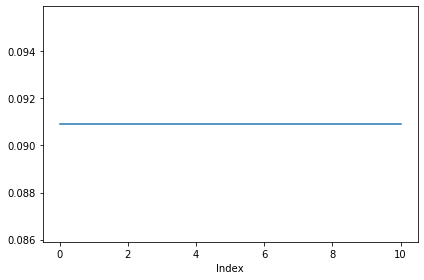Plot the wave. In : segment = wave.segment(duration=0.01) segment.plot() decorate(xlabel='Time (s)')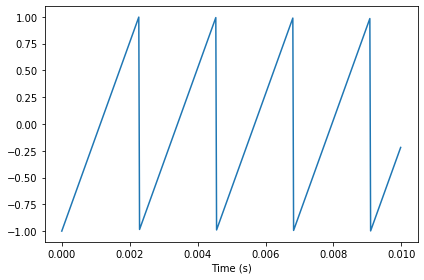Pad the window so it's the same length as the signal, and plot it. In : def zero_pad(array, n): """Extends an array with zeros. array: NumPy array n: length of result returns: new NumPy array """ res = np.zeros(n) res[:len(array)] = array return res  In : N = len(segment) padded = zero_pad(window, N) plt.plot(padded) decorate(xlabel='Index')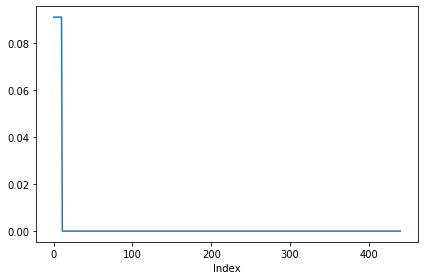Apply the window to the signal (with lag=0). In : prod = padded * segment.ys np.sum(prod)  Out: -0.9001814882032255 Compute a convolution by rolling the window to the right. In : smoothed = np.zeros(N) rolled = padded.copy() for i in range(N): smoothed[i] = sum(rolled * segment.ys) rolled = np.roll(rolled, 1)  In : plt.plot(rolled) decorate(xlabel='Index')Plot the result of the convolution and the original. In : from thinkdsp import Wave segment.plot(color='gray') smooth = Wave(smoothed, framerate=wave.framerate) smooth.plot() decorate(xlabel='Time(s)')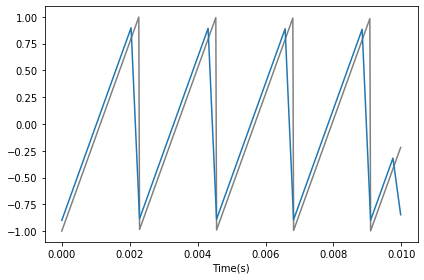Compute the same convolution using numpy.convolve. In : segment.plot(color='gray') ys = np.convolve(segment.ys, window, mode='valid') smooth2 = Wave(ys, framerate=wave.framerate) smooth2.plot() decorate(xlabel='Time(s)')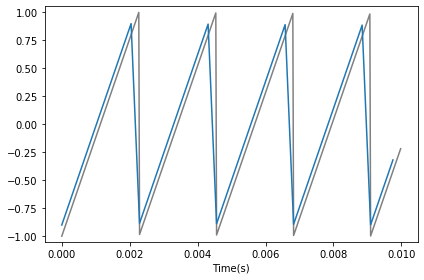## Frequency domain¶ Let's see what's happening in the frequency domain. Compute the smoothed wave using np.convolve, which is much faster than my version above. In : convolved = np.convolve(wave.ys, window, mode='same') smooth = Wave(convolved, framerate=wave.framerate) smooth.make_audio()  Out: Plot spectrums of the original and smoothed waves: In : spectrum = wave.make_spectrum() spectrum.plot(color='gray') spectrum2 = smooth.make_spectrum() spectrum2.plot() decorate(xlabel='Frequency (Hz)', ylabel='Amplitude')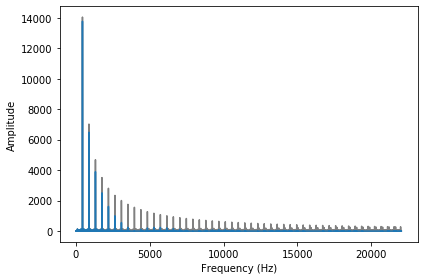For each harmonic, compute the ratio of the amplitudes before and after smoothing. In : amps = spectrum.amps amps2 = spectrum2.amps ratio = amps2 / amps ratio[amps<280] = 0 plt.plot(ratio) decorate(xlabel='Frequency (Hz)', ylabel='Amplitude ratio')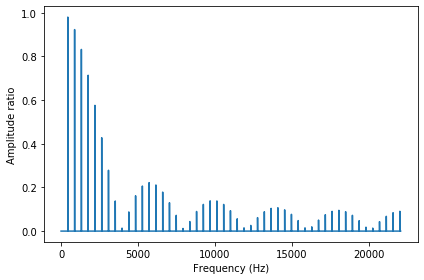Plot the ratios again, but also plot the FFT of the window. In : padded = zero_pad(window, len(wave)) dft_window = np.fft.rfft(padded) plt.plot(np.abs(dft_window), color='gray', label='DFT(window)') plt.plot(ratio, label='amplitude ratio') decorate(xlabel='Frequency (Hz)', ylabel='Amplitude ratio')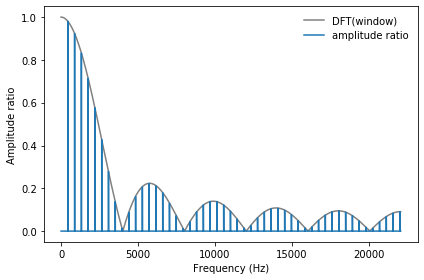### Gaussian window¶ Let's compare boxcar and Gaussian windows. Make the boxcar window. In : boxcar = np.ones(11) boxcar /= sum(boxcar)  Make the Gaussian window. In : import scipy.signal gaussian = scipy.signal.gaussian(M=11, std=2) gaussian /= sum(gaussian)  Plot the two windows. In : plt.plot(boxcar, label='boxcar') plt.plot(gaussian, label='Gaussian') decorate(xlabel='Index')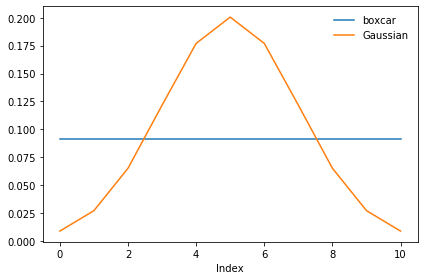Convolve the square wave with the Gaussian window. In : ys = np.convolve(wave.ys, gaussian, mode='same') smooth = Wave(ys, framerate=wave.framerate) spectrum2 = smooth.make_spectrum()  Compute the ratio of the amplitudes. In : amps = spectrum.amps amps2 = spectrum2.amps ratio = amps2 / amps ratio[amps<560] = 0  Compute the FFT of the window. In : padded = zero_pad(gaussian, len(wave)) dft_gaussian = np.fft.rfft(padded)  Plot the ratios and the FFT of the window. In : plt.plot(np.abs(dft_gaussian), color='0.7', label='Gaussian filter') plt.plot(ratio, label='amplitude ratio') decorate(xlabel='Frequency (Hz)', ylabel='Amplitude ratio')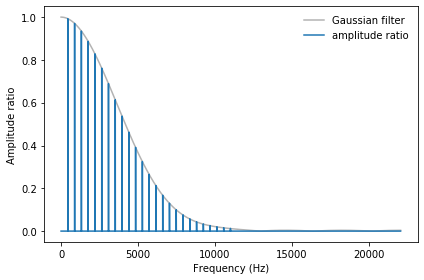Combine the preceding example into one big function so we can interact with it. In : from thinkdsp import SquareSignal def plot_filter(M=11, std=2): signal = SquareSignal(freq=440) wave = signal.make_wave(duration=1, framerate=44100) spectrum = wave.make_spectrum() gaussian = scipy.signal.gaussian(M=M, std=std) gaussian /= sum(gaussian) ys = np.convolve(wave.ys, gaussian, mode='same') smooth = Wave(ys, framerate=wave.framerate) spectrum2 = smooth.make_spectrum() # plot the ratio of the original and smoothed spectrum amps = spectrum.amps amps2 = spectrum2.amps ratio = amps2 / amps ratio[amps<560] = 0 # plot the same ratio along with the FFT of the window padded = zero_pad(gaussian, len(wave)) dft_gaussian = np.fft.rfft(padded) plt.plot(np.abs(dft_gaussian), color='gray', label='Gaussian filter') plt.plot(ratio, label='amplitude ratio') decorate(xlabel='Frequency (Hz)', ylabel='Amplitude ratio') plt.show()  Try out different values of M and std. In : from ipywidgets import interact, interactive, fixed import ipywidgets as widgets slider = widgets.IntSlider(min=2, max=100, value=11) slider2 = widgets.FloatSlider(min=0, max=20, value=2) interact(plot_filter, M=slider, std=slider2);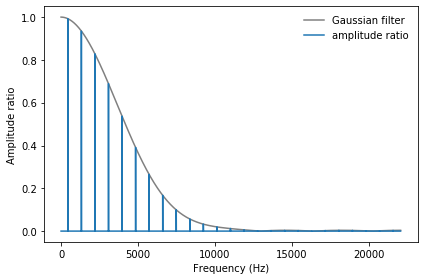## Convolution theorem¶ Let's use the Convolution theorem to compute convolutions using FFT. I'll use the Facebook data again, and smooth it using np.convolve and a 30-day Gaussian window. I ignore the dates and treat the values as if they are equally spaced in time. In : window = scipy.signal.gaussian(M=30, std=6) window /= window.sum() smoothed = np.convolve(close, window, mode='valid') len(close), len(smoothed)  Out: (1977, 1948) Plot the original and smoothed data. In : plt.plot(close, color='gray') plt.plot(smoothed) decorate(xlabel='Time (days)', ylabel='Price ($)')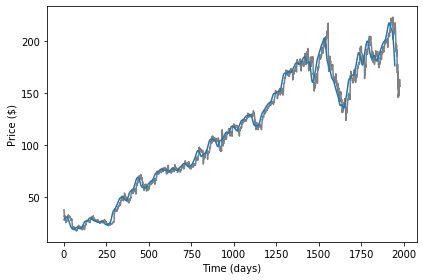Pad the window and compute its FFT.

In :
N = len(close)
plt.plot(np.abs(fft_window))
decorate(xlabel='Index')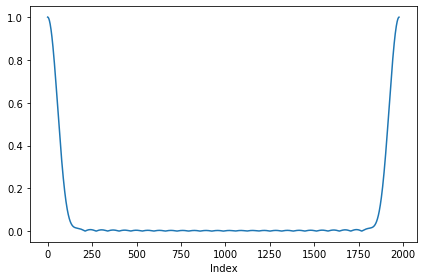Apply the convolution theorem.

In :
fft_signal = np.fft.fft(close)
smoothed2 = np.fft.ifft(fft_signal * fft_window)
M = len(window)
smoothed2 = smoothed2[M-1:]


Plot the two signals (smoothed with numpy and FFT).

In :
plt.plot(smoothed)
plt.plot(smoothed2.real)
decorate(xlabel='Time (days)', ylabel='Price (\$)')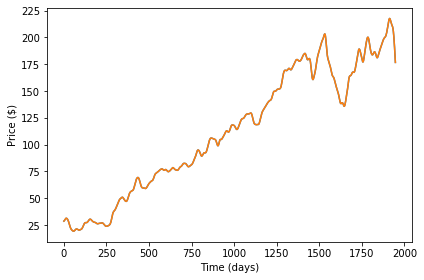Confirm that the difference is small.

In :
diff = smoothed - smoothed2
np.max(np.abs(diff))

Out:
2.089768879877275e-13

scipy.signal provides fftconvolve, which computes convolutions using FFT.

In :
smoothed3 = scipy.signal.fftconvolve(close, window, mode='valid')


Confirm that it gives the same answer, at least approximately.

In :
diff = smoothed - smoothed3
np.max(np.abs(diff))

Out:
8.526512829121202e-14

We can encapsulate the process in a function:

In :
def fft_convolve(signal, window):
fft_signal = np.fft.fft(signal)
fft_window = np.fft.fft(window)
return np.fft.ifft(fft_signal * fft_window)


And confirm that it gives the same answer.

In :
smoothed4 = fft_convolve(close, padded)[M-1:]
len(smoothed4)

Out:
1948
In :
diff = smoothed - smoothed4
np.max(np.abs(diff))

Out:
2.089768879877275e-13

### Autocorrelation¶

We can also use the convolution theorem to compute autocorrelation functions.

Compute autocorrelation using numpy.correlate:

In :
corrs = np.correlate(close, close, mode='same')
corrs[:7]

Out:
array([10515607.461, 10533254.812, 10550927.762, 10570279.165,
10589752.032, 10609151.979, 10628488.485])

Compute autocorrelation using my fft_convolve. The window is a reversed copy of the signal. We have to pad the window and signal with zeros and then select the middle half from the result.

In :
def fft_autocorr(signal):
N = len(signal)
window = np.flipud(signal)

corrs = fft_convolve(signal, window)
corrs = np.roll(corrs, N//2+1)[:N]
return corrs


Test the function.

In :
corrs2 = fft_autocorr(close)
corrs2[:7]

Out:
array([10515607.461-0.j, 10533254.812-0.j, 10550927.762+0.j,
10570279.165-0.j, 10589752.032+0.j, 10609151.979-0.j,
10628488.485-0.j])

Plot the results.

In :
lags = np.arange(N) - N//2
plt.plot(lags, corrs, color='gray', alpha=0.5, label='np.convolve')
plt.plot(lags, corrs2.real, color='C1', alpha=0.5, label='fft_convolve')
decorate(xlabel='Lag', ylabel='Correlation')
len(corrs), len(corrs2)

Out:
(1977, 1977)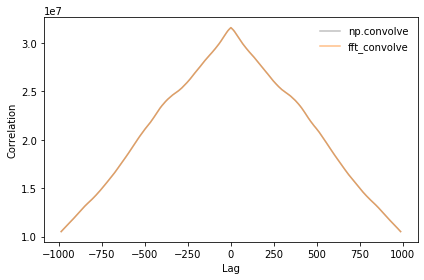Confirm that the difference is small.

In :
diff = corrs - corrs2.real
np.max(np.abs(diff))

Out:
2.60770320892334e-08
In [ ]: